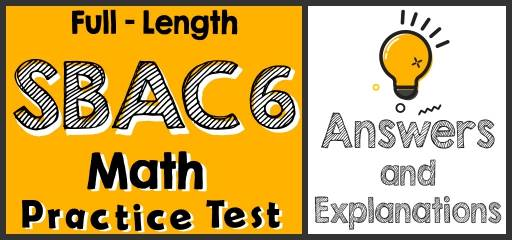Did you take the 6th Grade SBACMath Practice Test? If so, then it’s time to review your results to see where you went wrong and what areas you need to improve.

Plug in the value of $$x$$ and $$y$$ and use order of operations rule. $$x=2$$ and $$y=-3$$
$$5(4x-3y)-7y^2=5(4(2)-3(-3))-7(-3)^2=5(8+9)-7(9)=5(17)-63=85-63=22$$
For one hour he earns $18, then for t hours he earns$18t. If he wants to earn at least $78, therefore, the number of working hours multiplied by 18 must be equal to 78 or more than 78. $$18t≥78$$ 3- Choice B is correct $$(108-(3×9))÷9=9^3÷81=9$$ 4- Choice B is correct The ratio of boys to girls is 3 ∶ 5. Therefore, there are 3 boys out of 8 students. To find the answer, first, divide the total number of students by 8, then multiply the result by 3. $$240÷8=30 ⇒ 30×3=90$$ 5- Choice A is correct Probability$$=\frac{number \space of \space desired \space outcomes}{number \space of \space total \space outcomes}=\frac{9}{9+15+14+16}=\frac{9}{54}\frac{1}{6}=0.16$$ 6- Choice D is correct Let’s compare each fraction: $$\frac{2}{3}<\frac{3}{4}<\frac{7}{9}<\frac{4}{5}$$ Only choice D provides the right order. 7- Choice B is correct Let $$y$$ be the width of the rectangle. Then; $$14×y=84→y=\frac{84}{16}=6$$ 8- Choice B is correct $$4×\frac{5}{16}=\frac{20}{16}=1.25$$ A. $$1.25>2$$ B. $$1<1.25<2$$ This is the answer! C. $$\frac{3}{8}=1.25$$ D. $$1.25=2^2$$ 9- Choice B is correct In any rectangle, The measure of the sum of all the angles equals $$180^\circ$$. 10- Choice C is correct $$\frac{824}{17}=48.5$$ ## The Absolute Best Book to Ace the 6th Grade SBAC Math Test 11- The answer is $$7^2$$. $$588=2^2×3^1×7^2$$ 12- Choice B is correct The area of the trapezoid is: Area $$=\frac{base \space 1+base \space 2}{2}×height=\frac{12+10}{2}x=A→ 11x=A→x=\frac{A}{11}$$ 13- Choice B is correct $$\frac{72}{8}=9, \frac{648}{72}=9, \frac{5,832}{648}=9$$, Therefore, the factor is 9. 14- Choice C is correct Simplify each option provided. A. $$13-(3×6)+(7×(-6))=13-18+(-42)=-5-42=-47$$ B. $$(\frac{25}{400})+(\frac{7}{50})=\frac{25}{400}+\frac{56}{400}=\frac{81}{400}$$ C. $$((22×\frac{30}{6})-(7×\frac{144}{12}))×\frac{18}{2}=(110-84)×9=26×9=234$$ (this is the answer) D. $$(\frac{6}{24}+\frac{12}{33})-50=(\frac{1}{4}+\frac{1}{3})-50=(\frac{3}{12}+\frac{4}{12})-50=\frac{7}{12}-50=\frac{-593}{15}$$ 15- Choice D is correct To find the discount, multiply the number ($$100\%$$- rate of discount) Therefore; $$450(100\%-16\%)=450(1-0.16)=450-(450×0.16)$$ 16- Choice A is correct 1,400 out of 11,900 equals to $$\frac{1,400}{11,900}=\frac{200}{1,700}=\frac{2}{17}$$ 17- Choice C is correct The opposite of Nicolas’s integer is $$25$$. So, the integer is $$-25$$. The absolute value of $$25$$ is also $$25$$. 18- Choice B is correct Volume of a box = length × width × height = 7 × 4 × 12 = 336 19- Choice C is correct 1 yard = 3 feet, Therefore, $$33,759 ft×\frac{1 \space yd}{3 \space ft}=11,253$$ yd 20- Choice B is correct $$16\%$$ of the volume of the solution is alcohol. Let $$x$$ be the volume of the solution. Then: $$16\%$$ of $$x=38$$ ml ⇒ $$0.16x=38 ⇒ x=38÷0.16=237.5$$ ## Best 6th Grade SBAC Math Prep Resource for 2022 21- Choice C is correct $$(-2)(9x-8)=(-2)(9x)+(-2)(-8)=-18x+16$$ 22- Choice D is correct 1 pt = 16 fluid ounces. $$576÷16=36$$ Then: 576 fluid ounces = 36 pt 23- Choice D is correct 1 kg = 1000 g and 1 g = 1000 mg, 120 kg = 120 × 1000 g = 120 × 1000 × 1000 = 120,000,000 mg 24- Choice C is correct The diameter of a circle is twice the radius. Radius of the circle is $$\frac{14}{2}=7$$. Area of a circle $$= πr^2=π(7)^2=49π=49×3.14=153.86≅153.9$$ 25- Choice B is correct Average (mean) $$=\frac{sum \space of \space terms}{number \space of \space terms}=\frac{15+17+12+16+21+23}{6}=\frac{104}{6}=17.33$$ 26- Choice C is correct Prime factorizing of $$18=2×3×3$$, Prime factorizing of $$24=2×2×2×3$$ LCM $$= 2×2×2×3×3=72$$ 27- Choice B is correct The coordinate plane has two axes. The vertical line is called the $$y$$-axis and the horizontal is called the $$x$$-axis. The points on the coordinate plane are addressed using the form $$(x,y)$$. Point A is one unit on the left side of $$x$$-axis, therefore its $$x$$ value is 4 and it is two units up, therefore its $$y$$ axis is 2. The coordinate of the point is: (4, 2) 28- Choice B is correct $$α$$ and $$β$$ are supplementary angles. The sum of supplementary angles is 180 degrees. $$α+β=180^\circ→α=180^\circ-β=180^\circ-125^\circ=55^\circ,$$ Then, $$\frac{α}{β}=\frac{55}{125}=\frac{11}{25}$$ 29- Choice C is correct Opposite number of any number $$x$$ is a number that if added to $$x$$, the result is 0. Then: $$7+(-7)=0$$ and $$4+(-4)=0$$ 30- Choice C is correct $$16=-129+x$$, First, subtract 129 from both sides of the equation. Then: $$16+129=-129+x+129 →145=x$$ ## The Best Books to Ace the 6th Grade SBAC Math Test ## Related to This Article ### More math articles ### What people say about "Full-Length 6th Grade SBAC Math Practice Test-Answers and Explanations - Effortless Math: We Help Students Learn to LOVE Mathematics"? No one replied yet. X 21% OFF Limited time only! Save Over 21% SAVE$5
It was $23.99 now it is$18.99